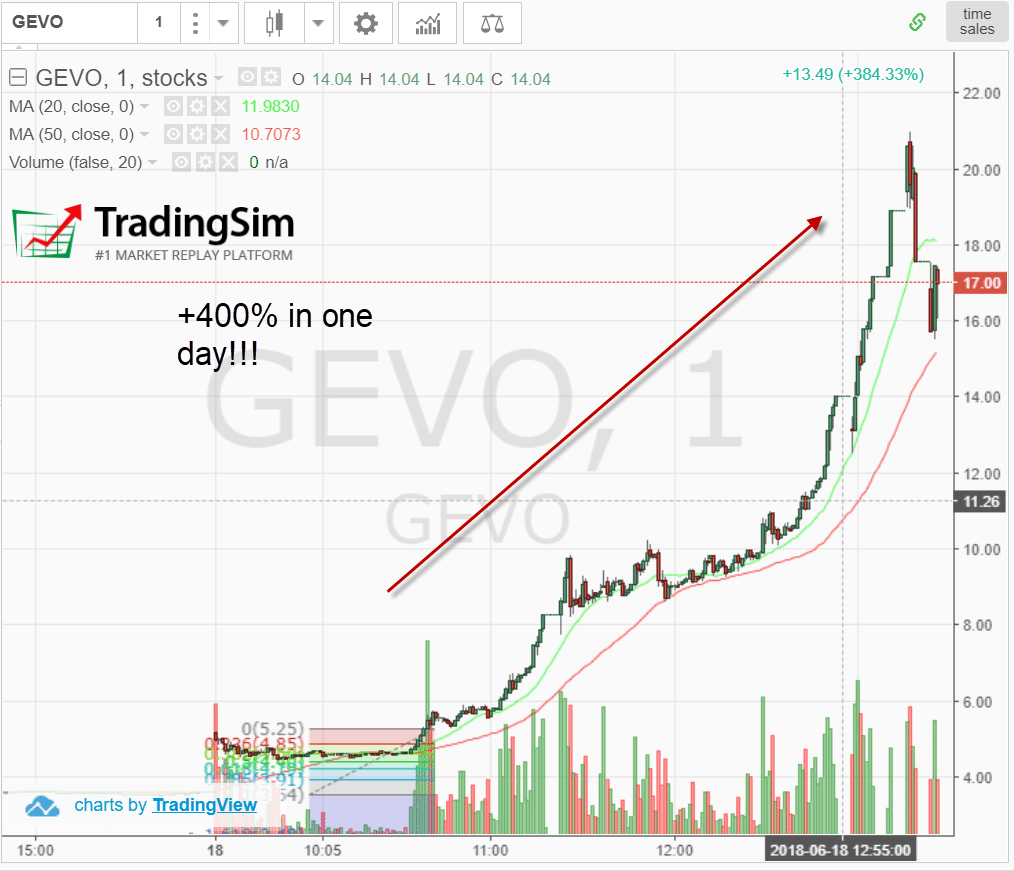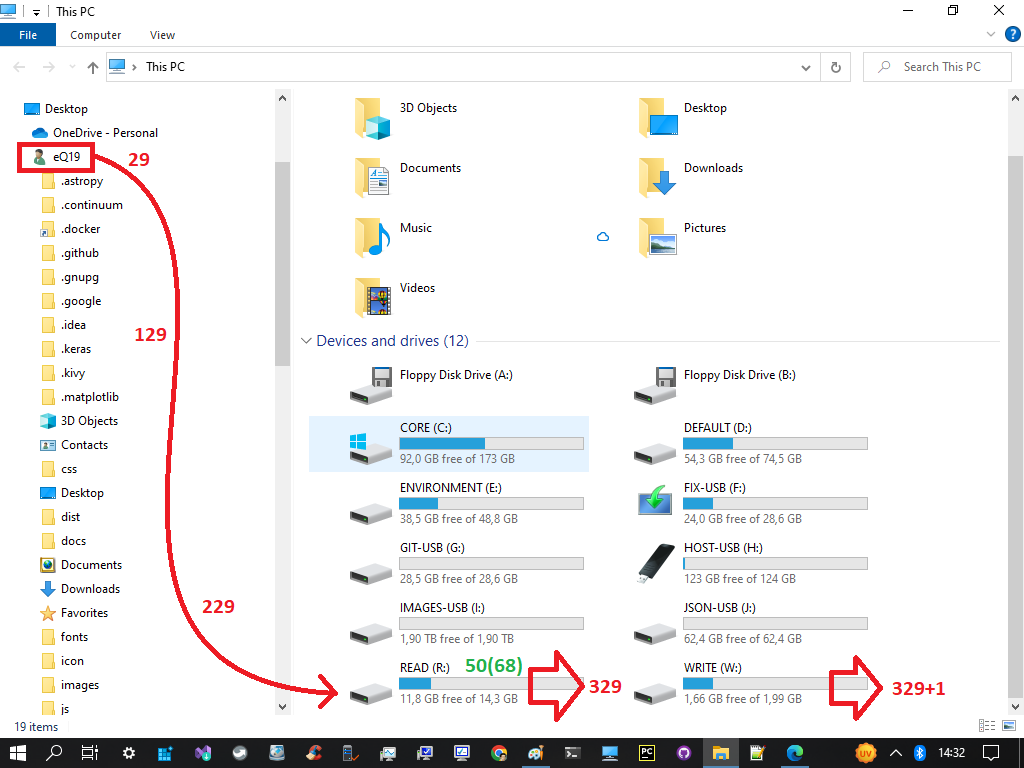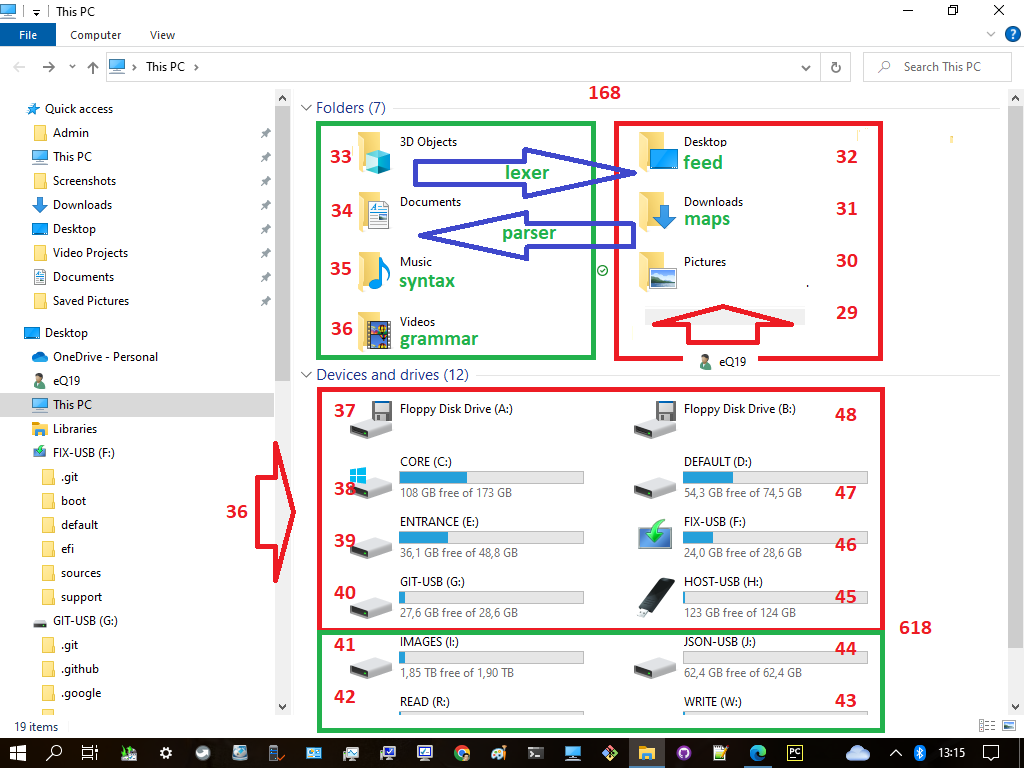``````1st layer:
It has a total of 1000 numbers
Total primes = π(1000) = 168 primes

2nd layer:
It will start by π(168)+1 as the 40th prime
It has 100x100 numbers or π(π(10000)) = 201 primes
Total cum primes = 168 + (201-40) = 168+161 = 329 primes

3rd layer:
Behave the same as 2nd layer which has a total of 329 primes
The primes will start by π(π(π(1000th prime)))+1 as the 40th prime
This 1000 primes will become 1000 numbers by 1st layer of the next level
Total of all primes = 329 + (329-40) = 329+289 = 618 = 619-1 = 619 primes - Δ1
``````

The 619 is the 114th prime. It has the last index of 6 with the prime 19 where as 6x19 is also 114. When we multiply the 114 minor hexagon again by six (6) minus the duplicaton within those layers we got 684-30-30-5 = 684-65 which is also 619.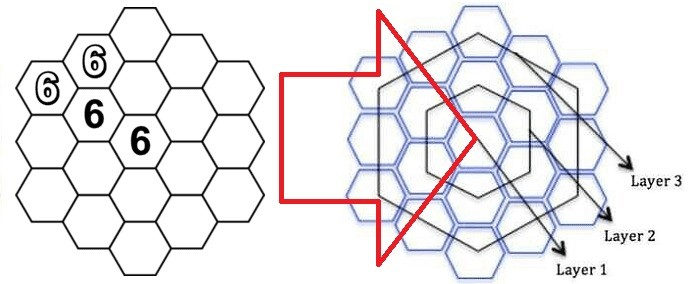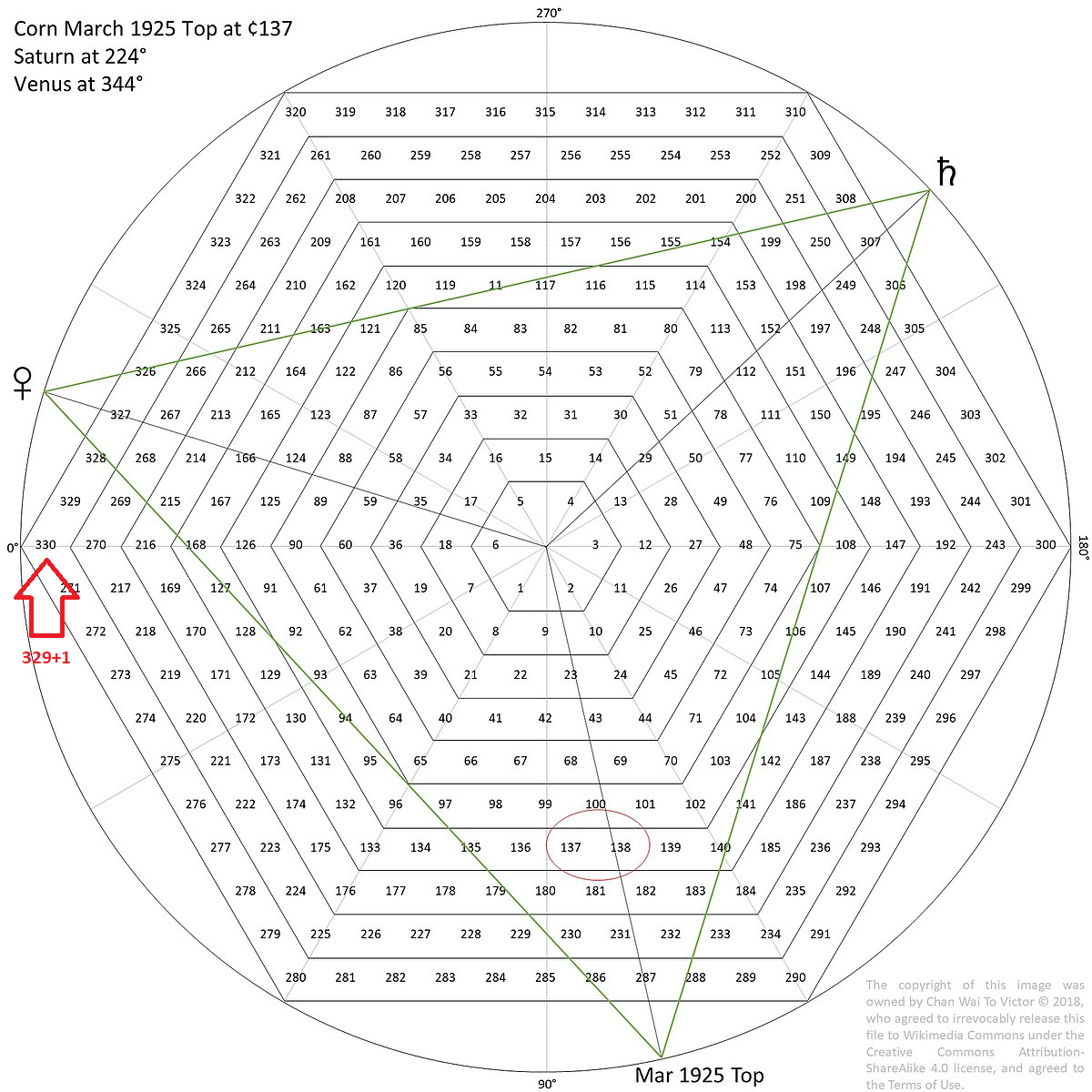Despite the correlation between the bilateral 9 sums with the Fibonacci sequence, some people has discovered many things. The sequence of (55, 89, 144) shows an almost mystical power by its mysterious accuracy in nature and even in trading.